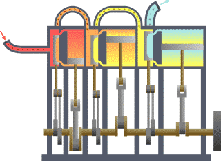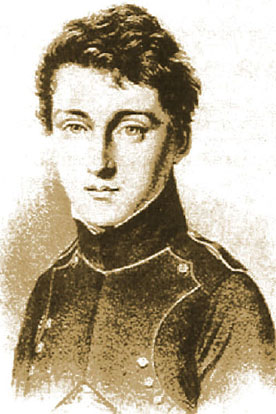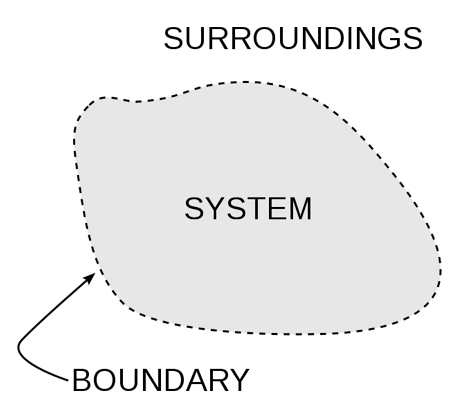ThermodynamicsBy Wikipedia,
the free encyclopedia,

http://en.wikipedia.org/wiki/Thermodynamics

In physics, thermodynamics (from the Greek θερμ-<θερμότης, therme, meaning "heat" and δυναμις, dynamis, meaning "power") is the study of the conversion of energy into work and heat and its relation to macroscopic variables such as temperature and pressure. Its underpinnings, based upon statistical predictions of the collective motion of particles from their microscopic behavior, is the field of statistical thermodynamics, a branch of statistical mechanics.. Historically, thermodynamics developed out of need to increase the efficiency of early steam engines.Typical thermodynamic system, showing input from a heat source (boiler) on the left and output to a heat sink (condenser) on the right. Work is extracted, in this case by a series of pistons.

## Starting point and relevance

The starting point for most thermodynamic considerations are the laws of thermodynamics, which postulate that energy can be exchanged between physical systems as heat or work. They also postulate the existence of a quantity named entropy, which can be defined for any system. In thermodynamics, interactions between large ensembles of objects are studied and categorized. Central to this are the concepts of system and surroundings. A system is composed of particles, whose average motions define its properties, which in turn are related to one another through equations of state. Properties can be combined to express internal energy and thermodynamic potentials, which are useful for determining conditions for equilibrium and spontaneous processes.

With these tools, the usage of thermodynamics describes how systems respond to changes in their surroundings. This can be applied to a wide variety of topics in science and engineering, such as engines, phase transitions, chemical reactions, transport phenomena, and even black holes. The results of thermodynamics are essential for other fields of physics and for chemistry, chemical engineering, aerospace engineering, mechanical engineering, cell biology, biomedical engineering, materials science, and economics to name a few.

## HistorySadi Carnot (1796-1832): was the father of thermodynamics

The history of thermodynamics as a scientific discipline generally begins with Otto von Guericke who, in 1650, built and designed the world's first vacuum pump and demonstrated a vacuum using his Magdeburg hemispheres. Guericke was driven to make a vacuum in order to disprove Aristotle's long-held supposition that 'nature abhors a vacuum'. Shortly after Guericke, the Irish physicist and chemist Robert Boyle had learned of Guericke's designs and, in 1656, in coordination with English scientist Robert Hooke, built an air pump. Using this pump, Boyle and Hooke noticed a correlation between pressure, temperature, and volume. In time, Boyle's Law was formulated, which states that pressure and volume are inversely proportional. Then, in 1679, based on these concepts, an associate of Boyle's named Denis Papin built a bone digester, which was a closed vessel with a tightly fitting lid that confined steam until a high pressure was generated.

Later designs implemented a steam release valve that kept the machine from exploding. By watching the valve rhythmically move up and down, Papin conceived of the idea of a piston and a cylinder engine. He did not, however, follow through with his design. Nevertheless, in 1697, based on Papin's designs, engineer Thomas Savery built the first engine. Although these early engines were crude and inefficient, they attracted the attention of the leading scientists of the time.

Their work led 127 years later to Sadi Carnot, the "father of thermodynamics", who, in 1824, published Reflections on the Motive Power of Fire, a discourse on heat, power, and engine efficiency. The paper outlined the basic energetic relations between the Carnot engine, the Carnot cycle, and Motive power. This marks the start of thermodynamics as a modern science.

The term thermodynamics was coined by James Joule in 1849 to designate the science of relations between heat and power. By 1858, "thermo-dynamics", as a functional term, was used in William Thomson's paper An Account of Carnot's Theory of the Motive Power of Heat. The first thermodynamic textbook was written in 1859 by William Rankine, originally trained as a physicist and a civil and mechanical engineering professor at the University of Glasgow.

## The laws of thermodynamics

In thermodynamics, there are four laws that do not depend on the details of the systems under study or how they interact. Hence these laws are very generally valid, can be applied to systems about which one knows nothing other than the balance of energy and matter transfer. Examples of such systems include Einstein's prediction, around the turn of the 20th century, of spontaneous emission, and ongoing research into the thermodynamics of black holes.

These four laws are:

If two thermodynamic systems are separately in thermal equilibrium with a third, they are also in thermal equilibrium with each other.
If we grant that all systems are (trivially) in thermal equilibrium with themselves, the Zeroth law implies that thermal equilibrium is an equivalence relation on the set of thermodynamic systems. This law is tacitly assumed in every measurement of temperature. Thus, if we want to know if two bodies are at the same temperature, it is not necessary to bring them into contact and to watch whether their observable properties change with time.
The change in the internal energy of a closed thermodynamic system is equal to the sum of the amount of heat energy supplied to the system and the work done on the system.
The total entropy of any isolated thermodynamic system tends to increase over time, approaching a maximum value.
As a system asymptotically approaches absolute zero of temperature all processes virtually cease and the entropy of the system asymptotically approaches a minimum value; also stated as: "the entropy of all systems and of all states of a system is zero at absolute zero" or equivalently "it is impossible to reach the absolute zero of temperature by any finite number of processes".

The following has sometimes been called the "Fourth Law of Thermodynamics", about the transfer of heat energy between systems.

In connected thermodynamic systems which are in equilibrium neither for pressure nor temperature, heat flow between is caused by forces proportional with unit of pressure difference, and equal to the proportional density flow caused per unit of temperature difference.
See also: Bose–Einstein condensate and negative temperature.

## Thermodynamic potentials

As can be derived from the energy balance equation (or Burks' equation) on a thermodynamic system there exist energetic quantities called thermodynamic potentials, being the quantitative measure of the stored energy in the system. The five most well known potentials are:

Other thermodynamic potentials can be obtained through Legendre transformation. Potentials are used to measure energy changes in systems as they evolve from an initial state to a final state. The potential used depends on the constraints of the system, such as constant temperature or pressure. Internal energy is the internal energy of the system, enthalpy is the internal energy of the system plus the energy related to pressure-volume work, and Helmholtz and Gibbs energy are the energies available in a system to do useful work when the temperature and volume or the pressure and temperature are fixed, respectively.

## Classical thermodynamics

Classical thermodynamics is the original early 1800s variation of thermodynamics concerned with thermodynamic states, and properties as energy, work, and heat, and with the laws of thermodynamics, all lacking an atomic interpretation. In precursory form, classical thermodynamics derives from chemist Robert Boyle’s 1662 postulate that the pressure P of a given quantity of gas varies inversely as its volume V at constant temperature; i.e. in equation form: PV = k, a constant. From here, a semblance of a thermo-science began to develop with the construction of the first successful atmospheric steam engines in England by Thomas Savery in 1697 and Thomas Newcomen in 1712. The first and second laws of thermodynamics emerged simultaneously in the 1850s, primarily out of the works of William Rankine, Rudolf Clausius, and William Thomson (Lord Kelvin).

## Statistical mechanics

With the development of atomic and molecular theories in the late 1800s and early 1900s, thermodynamics was given a molecular interpretation. This field, called statistical mechanics or statistical thermodynamics, relates the microscopic properties of individual atoms and molecules to the macroscopic or bulk properties of materials that can be observed in everyday life, thereby explaining thermodynamics as a natural result of statistics and mechanics (classical and quantum) at the microscopic level. The statistical approach is in contrast to classical thermodynamics, which is a more phenomenological approach that does not include microscopic details. The foundations of statistical thermodynamics were set out by physicists such as James Clerk Maxwell, Ludwig Boltzmann, Max Planck, Rudolf Clausius and J. Willard Gibbs.

## Chemical thermodynamics

Chemical thermodynamics is the study of the interrelation of energy with chemical reactions or with a physical change of state within the confines of the laws of thermodynamics. During the years 1873-76 the American mathematical physicist Josiah Willard Gibbs published a series of three papers, the most famous being On the Equilibrium of Heterogeneous Substances, in which he showed how thermodynamic processes could be graphically analyzed, by studying the energy, entropy, volume, temperature and pressure of the thermodynamic system, in such a manner to determine if a process would occur spontaneously. During the early 20th century, chemists such as Gilbert N. Lewis, Merle Randall, and E. A. Guggenheim began to apply the mathematical methods of Gibbs to the analysis of chemical processes.

## Thermodynamic systemsAn important concept in thermodynamics is the “system”. Everything in the universe except the system is known as surroundings. A system is the region of the universe under study. A system is separated from the remainder of the universe by a boundary which may be imaginary or not, but which by convention delimits a finite volume. The possible exchanges of work, heat, or matter between the system and the surroundings take place across this boundary. Boundaries are of four types: fixed, moveable, real, and imaginary.

Basically, the “boundary” is simply an imaginary dotted line drawn around a volume of something when there is going to be a change in the internal energy of that something. Anything that passes across the boundary that effects a change in the internal energy of the something needs to be accounted for in the energy balance equation. That something can be the volumetric region surrounding a single atom resonating energy, such as Max Planck defined in 1900; it can be a body of steam or air in a steam engine, such as Sadi Carnot defined in 1824; it can be the body of a tropical cyclone, such as Kerry Emanuel theorized in 1986 in the field of atmospheric thermodynamics; it could also be just one nuclide (i.e. a system of quarks) as some are theorizing presently in quantum thermodynamics.

For an engine, a fixed boundary means the piston is locked at its position; as such, a constant volume process occurs. In that same engine, a moveable boundary allows the piston to move in and out. For closed systems, boundaries are real while for open system boundaries are often imaginary. There are five dominant classes of systems:

1. Isolated Systems – matter and energy may not cross the boundary
2. Adiabatic Systems – heat must not cross the boundary
3. Diathermic Systems - heat may cross boundary
4. Closed Systems – matter may not cross the boundary
5. Open Systems – heat, work, and matter may cross the boundary (often called a control volume in this case)

As time passes in an isolated system, internal differences in the system tend to even out and pressures and temperatures tend to equalize, as do density differences. A system in which all equalizing processes have gone practically to completion, is considered to be in a state of thermodynamic equilibrium.

In thermodynamic equilibrium, a system's properties are, by definition, unchanging in time. Systems in equilibrium are much simpler and easier to understand than systems which are not in equilibrium. Often, when analysing a thermodynamic process, it can be assumed that each intermediate state in the process is at equilibrium. This will also considerably simplify the situation. Thermodynamic processes which develop so slowly as to allow each intermediate step to be an equilibrium state are said to be reversible processes.

## Conjugate variables

The central concept of thermodynamics is that of energy, the ability to do work. By the First Law, the total energy of a system and its surroundings is conserved. Energy may be transferred into a system by heating, compression, or addition of matter, and extracted from a system by cooling, expansion, or extraction of matter. In mechanics, for example, energy transfer equals the product of the force applied to a body and the resulting displacement.

Conjugate variables are pairs of thermodynamic concepts, with the first being akin to a "force" applied to some thermodynamic system, the second being akin to the resulting "displacement," and the product of the two equalling the amount of energy transferred. The common conjugate variables are:

## Thermodynamic instruments

There are two types of thermodynamic instruments, the meter and the reservoir. A thermodynamic meter is any device which measures any parameter of a thermodynamic system. In some cases, the thermodynamic parameter is actually defined in terms of an idealized measuring instrument. For example, the zeroth law states that if two bodies are in thermal equilibrium with a third body, they are also in thermal equilibrium with each other. This principle, as noted by James Maxwell in 1872, asserts that it is possible to measure temperature. An idealized thermometer is a sample of an ideal gas at constant pressure. From the ideal gas law PV=nRT, the volume of such a sample can be used as an indicator of temperature; in this manner it defines temperature. Although pressure is defined mechanically, a pressure-measuring device, called a barometer may also be constructed from a sample of an ideal gas held at a constant temperature. A calorimeter is a device which is used to measure and define the internal energy of a system.

A thermodynamic reservoir is a system which is so large that it does not appreciably alter its state parameters when brought into contact with the test system. It is used to impose a particular value of a state parameter upon the system. For example, a pressure reservoir is a system at a particular pressure, which imposes that pressure upon any test system that it is mechanically connected to. The Earth's atmosphere is often used as a pressure reservoir.

It is important that these two types of instruments are distinct. A meter does not perform its task accurately if it behaves like a reservoir of the state variable it is trying to measure. If, for example, a thermometer were to act as a temperature reservoir it would alter the temperature of the system being measured, and the reading would be incorrect. Ideal meters have no effect on the state variables of the system they are measuring.

## Thermodynamic states

When a system is at equilibrium under a given set of conditions, it is said to be in a definite state. The state of the system can be described by a number of intensive variables and extensive variables. The properties of the system can be described by an equation of state which specifies the relationship between these variables. State may be thought of as the instantaneous quantitative description of a system with a set number of variables held constant.

## Thermodynamic processes

A thermodynamic process may be defined as the energetic evolution of a thermodynamic system proceeding from an initial state to a final state. Typically, each thermodynamic process is distinguished from other processes, in energetic character, according to what parameters, as temperature, pressure, or volume, etc., are held fixed. Furthermore, it is useful to group these processes into pairs, in which each variable held constant is one member of a conjugate pair. The seven most common thermodynamic processes are shown below:

1. An isobaric process occurs at constant pressure.
2. An isochoric process, or isometric/isovolumetric process, occurs at constant volume.
3. An isothermal process occurs at a constant temperature.
4. An adiabatic process occurs without loss or gain of energy by heat.
5. An isentropic process (reversible adiabatic process) occurs at a constant entropy.
6. An isenthalpic process occurs at a constant enthalpy.
7. A steady state process occurs without a change in the internal energy of a system.

## See also

### Other

Lists and timelines:

Thermodynamic:

Other Related Topics

## External links

Text from Wikipedia is available under the Creative Commons Attribution/Share-Alike License; additional terms may apply.

Published - July 2009

Please see some ads intermixed with other content from this site:Copyright 2004-2021 © by Airports-Worldwide.com, Vyshenskoho st. 36, Lviv 79010, Ukraine
Legal Disclaimer